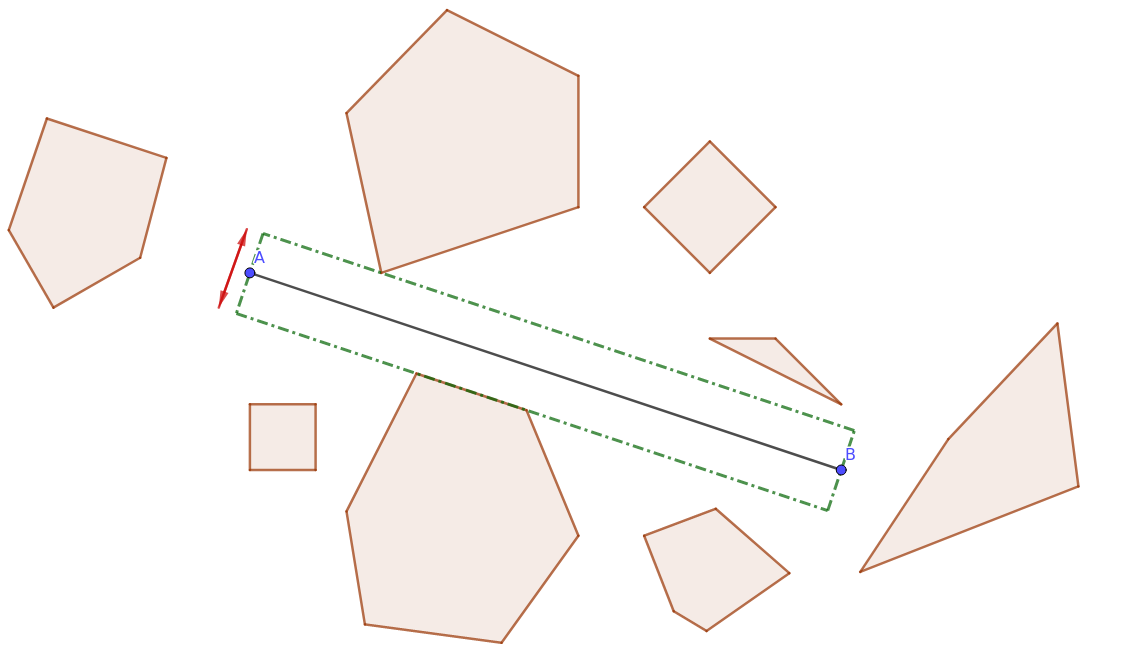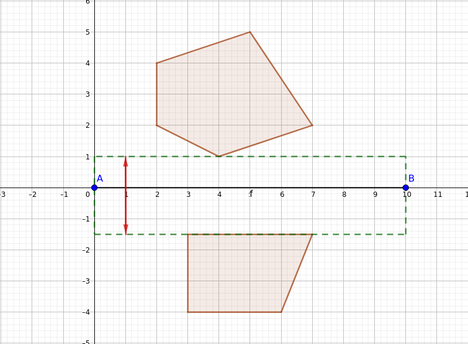Practice on Toph

Participate in exhilarating programming contests, solve unique algorithm and data structure challenges and be a part of an awesome community.

Expansion

By reborn · Limits 1s, 256 MB

The use of the central road in Utopia’s capital has increased by a great value in recent years. So, the Ministry of Transport wants to expand its width while keeping the length constant. However, there are important buildings on both sides of the highway and before requesting the Ministry of Habitation to move any of those, they want to find out the maximum width the road can have without intersecting the buildings.

Formally, there are $n$ buildings of convex polygonal shape and a line segment $AB$ representing the road. Initially, the width of the road is considered to be zero. The Ministry of Transport wants to find out the maximum width the road can have such that it does not go through any of the buildings i.e. no building should have a positive area on the road. After expansion, the road will be of a rectangular shape with two sides parallel to $AB$.In the example image above, there are 8 buildings at various locations. The road is depicted by the line segment $AB$. The expanded road is denoted via the green dotted rectangle. Note that, no building intersects the expanded road.

As the intern programmer at the Ministry of Transport, you have to find out the maximum width the central road can have.

Input

The first line contains a single positive integer $T$ denoting the number of test cases. Description of $T$ test cases follows. For each test case —

The first line contains four space-separated real numbers $A_x, A_y, B_x, B_y$ denoting the road endpoints $A = (A_x, A_y)$ and $B = (B_x, B_y)$, respectively.

The following line contains a positive integer $n$ denoting the number of convex polygons i.e. buildings.

$n$ lines follow, each describing a polygon. Each of these lines contain a positive integer $k$ denoting the number of vertices in the current polygon $P$ followed by $2k$ real numbers $P_{1x}, P_{1y}, P_{2x}, P_{2y}, P_{3x}, P_{3y}, \dots P_{kx}, P_{ky}$, denoting the coordinates of the vertices of the polygon.

Constraints

It is guaranteed that —

• $2 \le n \le 100$.

• $1 \le T \le 100$.

• The number of vertices of a polygon is inclusively between 3 and 10.

• For any coordinate $(x, y)$ in the input, $0 \le |x, y| \le 10^9$ and $x, y$ both have exactly two digits after the decimal point.

• In the input, no polygon touches or intersects with the given line segment, i.e. no part of the line is inside or on any polygon.

• The polygons are convex, have a positive area, and do not intersect among themselves.

• It is guaranteed that the maximum expanded width of the road is finite.

Output

Output a real number that denotes the maximum width the road can be expanded to. Your output will be considered correct if the absolute or relative error is less than $10^{-4}$.

Sample

InputOutput
1
0.00 0.00 10.00 0.00
2
5 2.00 2.00 4.00 1.00 7.00 2.00 5.00 5.00 2.00 4.00
4 3.00 -4.00 6.00 -4.00 7.00 -1.50 3.00 -1.50
2.50

Sample I/O ExplanationNotes

A convex polygon is a simple polygon where each of the interior angles is less than or equal to 180 degrees.

A simple polygon is a polygon that does not intersect itself and has no holes.

A polygon is a plane figure that is described by a finite number of straight-line segments connected to form a closed polygonal chain (or polygonal circuit).

A polygonal chain is a connected series of line segments.

Statistics

0% Solution Ratio

Submit